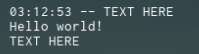# Need help with string.gsub (Replacing String)

Hey so I’m trying to replace a string using string.gsub but I’m getting this error and I would appreciate it if you can help me out.

``````local a="\\[\\[]]"
a = (a):gsub("%\\[","[")
print(a)
``````

Error I get when compiling.
This happens on Roblox and online Lua compilers.

``````--[[
Lua: main.lua:2: malformed pattern (missing ']')
stack traceback:
[C]: in function 'string.gsub'
main.lua:2: in main chunk
[C]: in ?
--]]
``````

I’m very confused since it works when I do this.

``````local a="[[\\]\\]"
a = (a):gsub("%\\]","]")
print(a)
-- Output: [[]]
``````

All I want to do is to replace anything that equals `"\]"` with `"]"`

Sincerely,
Joe

Every “[” requires an “]” to negate it, I have no idea why, I just know it creates raw strings, but you would use something like this:

``````local a= "\\[\\[]]"
a = a:gsub("%\\[[]", "]")
print(a)``````
1 Like

use `%` before typing `[` so that it’ll search for the character `[`

``````local a = "\\[\\[]]"
a = (a):gsub("%\\%[","[")
print(a)

-- output: [[]]
``````

Thanks for the help guys I appreciate it very much!
@Kaid3n22
@KING_CHYVZ

1 Like

The `[[` operator is used to start multi-line strings.

``````local LongString = [[TEXT HERE
Hello world!
TEXT HERE]]

print(LongString)
``````